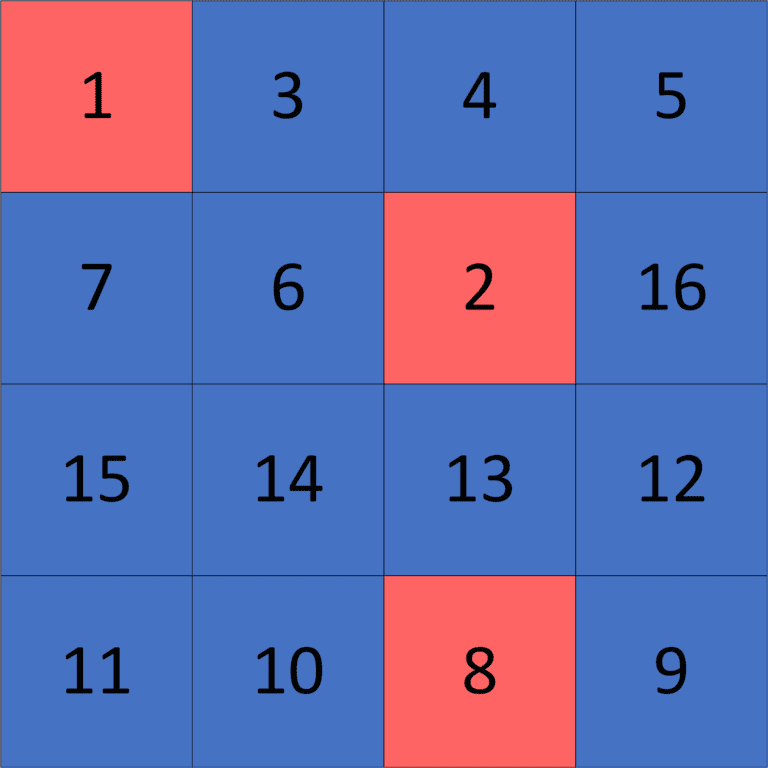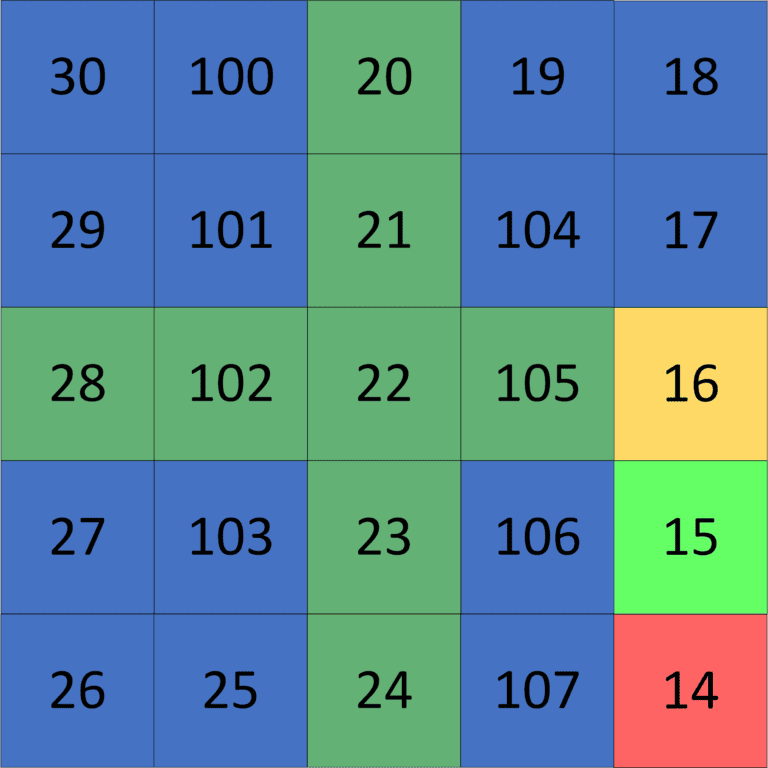## 1. Overview

In this tutorial, we’ll discuss the problem of finding the local minimum in axmatrix.

First, we’ll define the problem and provide an example that explains it.

Second, we’ll present a naive approach and then improve it to obtain a better solution.

## 2. Defining the Problem

Suppose we have amatrix of sizexthat consists ofxdistinct integers. Our task is to find a local minimum in the given matrix.

Recall that a local minimum in amatrix is the cell that has a value smaller than all its adjacent neighboring cells.

A cell has four adjacent neighboring cells if it’s inside the matrix. Furthermore, it has three adjacent neighboring cells if it’s on the border of the matrix. Finally, it has only two adjacent neighboring cells if positioned on any corner of the matrix.

Let’s check an example for better understanding. Suppose we have the followingmatrix of sizex:As we can see, there are three local minimum cells in our example:

1., because all of its two adjacent neighboring cells have a value greater than.
2., because all of its four adjacent neighbor cells have a value greater than.
3., because all of its three adjacent neighbor cells have a value greater than.

Therefore, the answer to our example could be any cell of these three local minimum cells.

## 3. Naive Approach

### 3.1. Main Idea

The main idea here is to always move to the smallest adjacent neighbor cell with a value smaller than the current cell value until we reach a local minimum.

To iterate over our matrix, we’ll use DFS (Depth First Search). Each time we reach a cell, we’ll check whether it’s a local minimum, which means that we’re done because we found a local minimum in the givenmatrix. Otherwise, we’ll move to the cell with the smallest value among all the adjacent cells that have a value smaller than the current cell value.

Eventually, this must terminate because moving to a cell with a value smaller than the current cell value cannot go on forever.

### 3.2. Implementation

Let’s take a look at the implementation:Initially, we declare thefunction that will return the smallest adjacent neighbor cell with a value smaller than the current cell value. For each cell, there are four possible adjacent cells we have to check.

First, we’ll declareandvalues representing the current row () and the current column (), respectively. Then we declare, which represents the value of the smallest adjacent cell. Initially, we assign the value of the current cell to it. Finally, we declare the, which will represent the smallest adjacent cell. To begin, it’ll be equal to thecell.

Next we’ll check whether the current row index is greater than, which means a cell above theone. Then we’ll check whether that cell’s value is smaller than the minimum value that we got so far. If so, we’ll update theto be equal to the cell located above thecell. We do the same process for the left, right, and down adjacent cells.

In the end, we return thethat we’ll move to in the next DFS traversal.

Then we declare thefunction, which returns the local minimum in the given matrix. To start, we get thethat we’ll move to in the next DFS call. Second, we’ll check whetherequals thecell, which means that thecell is a local minimum because it has no adjacent cell that has a value smaller than the value of thecell. Otherwise, we move to thein our DFS traversal.

### 3.3. Complexity

The complexity of this approach is the same as the DFS complexity, which is, whereis the number of cells andis the number of transitions. Thus, it’sbecause the number of cells is, while the number of transitions is a little less than. The reason behind this complexity is that we’ll iterate over each cell once in the worst-case scenario. Therefore, we iterate over its adjacent neighbors. In total, we iterate over each transition once as well.

## 4. Divide-and-Conquer Approach

### 4.1. Main Idea

We’ll divide the given matrix into four quadrant sub-matrices. We can guarantee that our local minimum will be inside one of these four sub-matrices. To find in which sub-matrix our local minimum exists, we’ll iterate over theand theto get the cell that has the smallest value among all of the values in theand the.

After we get the cell with the smallest value, we’ll get the(smallest adjacent cell) of it. Now the sub-matrix that has thewill also have the local minimum in it. This is true because if the local minimum is in another sub-matrix, it means we have to cross theor the, and that’s impossible because we’ve picked the cell that has the smallest value among all theand thecells.

Let’s take a look at the following example for better understanding:We take, which has the smallest value among all theand thecells. Then we get theof it which is. So we can guarantee that the local minimum will be inside thesub-matrix. Therefore, we solve our problem for thesub-matrix and repeat the same process.

### 4.2. Implementation

Let’s take a look at the implementation:Initially, we declare thefunction that’ll take the given matrix and return a local minimum cell in it.

Then we declare the indices of theand. After that, we declare the, representing the cell that has the smallest value among all the cells that lay in theand. To do that, we use the, andfunctions that return the minimum value in a certain row or column, respectively.

We’ll get theof theas we did in the previous approach to know in which sub-matrix our local minimum is located.

Now we’ll check if theis equal to the. In this case, it means theis a local minimum, so we return it and finish the algorithm at this point.

However, if theis less than the, it means that our local minimum is either in the Top-Left or Top-Right sub-matrices. Therefore, we have to check whether theis less than, meaning we have a local minimum in the Top-Left sub-matrix. Otherwise, it’ll be in the Top-Right sub-matrix.

Finally, we’ll check if theis greater than or equal to the, which means that our local minimum is either in the Bottom-Left or Bottom-Right sub-matrices. We check it in the same manner as we did in the previous conditions.

### 4.3. Complexity

The complexity here is. Let’s examine the reason behind this complexity.

In the first call, we iterate overcells to get the. However, in the second call, we iterate overcells. After that, we iterate overcells, and so on.

So the total number of iterations will be equal to, which means the complexity is.

## 5. Conclusion

In this article, we presented the problem of finding a local minimum in amatrix. We explained the general idea and discussed two approaches.

First, we explained the naive one. Then we improved it to get theapproach, which was much faster than the first one.